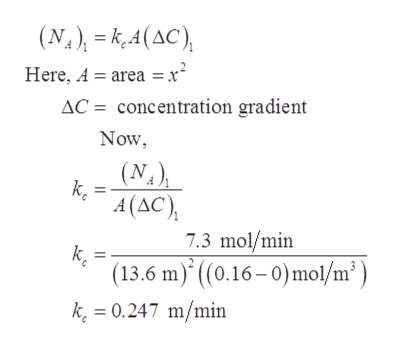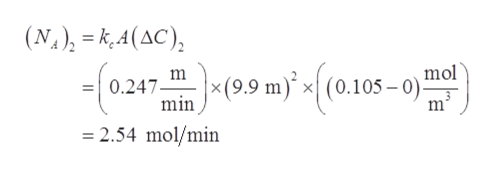# Some discarded solid chemical waste dissolves slowly ina large drain pipe in which the water is stagnant. On a particularday, the dissolved chemical has a concentration of 0.16 Mnear the solid and is essentially 0 at a location 13.6 m furtheralong the pipe. The transfer rate (moles per time) of the chemicalthrough the water in the pipe between those two points is7.3 gmol/min.a. What equation describes this kind of transfer?b. Several days later, the chemical concentration near thesolid has decreased to 0.105 M and is essentially 0 ata location 9.9 m away. What will be the transfer ratebetween the point near the solid and the point 9.9 maway on this later day?c. On the next day, the heavy rains cause a current of waterto flow through the drain pipe where the dissolving solidis located. The solid now dissolves twice as fast as onthe previous day. If the concentrations are still 0.105 Mnear the solid and 0 at the more remote locations, andif the cross-sectional area for transfer is 0.3 m2, what isthe value of the mass-transfer coefficient at this time?

Question
23 views

Some discarded solid chemical waste dissolves slowly in
a large drain pipe in which the water is stagnant. On a particular
day, the dissolved chemical has a concentration of 0.16 M
near the solid and is essentially 0 at a location 13.6 m further
along the pipe. The transfer rate (moles per time) of the chemical
through the water in the pipe between those two points is
7.3 gmol/min.
a. What equation describes this kind of transfer?
b. Several days later, the chemical concentration near the
solid has decreased to 0.105 M and is essentially 0 at
a location 9.9 m away. What will be the transfer rate
between the point near the solid and the point 9.9 m
away on this later day?
c. On the next day, the heavy rains cause a current of water
to flow through the drain pipe where the dissolving solid
is located. The solid now dissolves twice as fast as on
the previous day. If the concentrations are still 0.105 M
near the solid and 0 at the more remote locations, and
if the cross-sectional area for transfer is 0.3 m2, what is
the value of the mass-transfer coefficient at this time?

check_circle

Step 1

(a)

In the given problem, there is diffusion of moles occurring over time. Therefore, molecular diffusion equation describes this kind of transfer.

Step 2

(b)

Let,

Dissolved chemical at point 1 be represented by ‘(CA)’.

Dissolved chemical at point 2 be represented by ‘(C0)’.

Distance between two points of dissolved chemical be represented by ‘x’.

Transfer rate be represented by ‘NA’.

Mass transfer coefficient is represented by ‘kc’.

For a particular day, the data given are:

(CA)1 = 0.16 M

(C0)1 = 0 M

x1 = 13.6 m

(NA)1 = 7.3 gmol/min

From this given data, calculate the value of mass transfer coefficient, kc as:help_outlineImage Transcriptionclose(N.), = k,4(AC), Here, A = area = x² AC = concentration gradient Now, (N.), k. A (AC), 7.3 mol/min k. (13.6 m)* ((0.16– 0)mol/m²) k. = 0.247 m/min fullscreen
Step 3

After several days, the concentration of dissolved chemical is:

(CA)2 = 0.105M

(C0)2 = 0 M

x2 = 9.9 m

The value of mass transfer ...help_outlineImage Transcriptionclose(N.), = k,A(AC), x(9.9 m) %3D -(0247 199 m)' - (0.105-0) mol 05 – 0) m 0.247. min %3D = 2.54 mol/min fullscreen

### Want to see the full answer?

See Solution

#### Want to see this answer and more?

Solutions are written by subject experts who are available 24/7. Questions are typically answered within 1 hour.*

See Solution
*Response times may vary by subject and question.
Tagged in

### Mass Transfer